## Introduction

The discovery of the integer quantum Hall effect in field-effect transistors1 initiated a novel approach to the electronic properties of crystalline solids based on the topological properties of Bloch states2, which fostered the discovery of further remarkable effects, such as the fractional quantum Hall effect3, the quantum spin Hall effect4 and charge antilocalization5,6. These effects suggest novel device concepts for quantum computation and spintronics, where information is carried without dissipation by using spin, instead of charge, currents7. In this context, there has been an increasing interest in systems where the spin-orbit (SO) coupling leads to the opening of gaps at the Fermi surface and to spin-polarized bands8. An attractive possibility is the splitting of electronic states with opposite spin chirality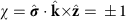in nonmagnetic materials by the SO Rashba effect. The Rashba Hamiltonian,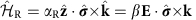, where and β are constants andis the spin vector operator composed of the Pauli matrices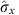,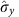and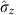, requires an electric field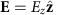that breaks the inversion symmetry along the z-direction perpendicular to the plane containing the spin σ and the electron wave vector k.

It follows that typical Rashba systems are either bulk noncentrosymmetric crystals or surfaces, referred to as bulk or surface inversion asymmetry systems, respectively8. Notable examples are the layered polar semiconductor BiTeI9 and the two-dimensional electron gas formed at the surface of SrTiO3 (ref. 10), where Rashba splittings as large as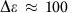meV have been reported. These large splittings are due to the presence of heavy elements (and thus to large SO couplings) in the former case and to a large electric field Ez≈10 mV Å−1 at the surface in the latter case. In fact, as noted recently by Zhang et al.11, SO coupling effects are governed by the local symmetry of the potential felt by the electron, rather than by the symmetry of the bulk crystal. To the best of our knowledge, the first experimental evidence of this circumstance has been given by Riley et al.12 who observed spin-polarized bands in the layered system 2H-WSe2. This polarization, not expected in the centrosymmetric P63/mmc structure of 2H-WSe2, is explained by the confinement of the electron states within a single WSe2 layer with local inversion asymmetry (LIA)13.

In the present work, we consider the quasi-two-dimensional semi-metal BaNiS2 (ref. 14), precursor of the metal–insulator transition (MIT) observed in the BaCo1−xNixS2 system on the partial substitution of Ni for Co at xcr=0.22 (ref. 15). This MIT has attracted a great deal of interest for the similarity of its temperature-doping (T-x) phase diagram to that of cuprate16, pnictide17 and heavy-fermion18 superconductors, though it remains little studied. To the best of our knowledge, the experimental band structure is not available for BaNiS2 and first-principles calculations have been hitherto limited to a simple density functional theory (DFT) approach in the local density approximation neglecting the Hubbard repulsion term, U, and relativistic SO effects19,20. The strong local Coulomb repulsion is expected to play a crucial role on the MIT, as indicated by dynamical mean field theory calculations on the insulating phase BaCoS2 (refs 21, 22). To elucidate these open issues, the objective of the present study is to provide a first thorough description of the electronic structure of BaNiS2. Previous calculations show that one challenge is a complex multiband structure. On the other hand, a favourable condition of this study is the absence of disorder caused by chemical substitutions and a simple tetragonal structure. By means of angular-resolved photoemission spectroscopy (ARPES) on high-quality single crystals, we find a very large Rashba splitting up to Δɛ≈150 meV, which is unexpected in a centrosymmetric system without electronically active heavy elements. This observation is explained by a very effective LIA mechanism associated with a peculiar non-symmorphic square-pyramidal structure with a gliding plane symmetry. Unlike WSe2, in the present case, the large splittings observed are not due to the presence of heavy elements but to a huge staggered crystal field at the Ni site, Ez≈1.4 eV Å−1, associated with the pyramidal coordination of the Ni ions. The mechanism unveiled by the present results, therefore, opens new possibilities to tune effectively the topological order of solids without the constraint of using either heavy elements or external fields.

## Results

### Ab initio calculations

Before presenting the ARPES results and the evidence of Rashba effect, we recall the salient features of the electronic structure of BaNiS2 that we recalculated in the generalized gradient approximation (GGA) of DFT supplemented by the Hubbard repulsion, U, and by the Hund coupling, J. In Fig. 1a–c, we show the crystal structure, the calculated Fermi surface, the band structure and the total and projected density of states (DOS) for each d-orbital of Ni. The parameters of the local interaction matrix (U=3 eV, J=0.95 eV) were estimated from absorption data23. In the band structure of Fig. 1c, false colours represent the d-orbital component of each band. We use a reference system for the d-orbitals where the x (y) axis coincides with the a (b) axis, while the z axis coincides with the c axis and points towards the apical sulphur atom. All d-orbitals, except the low-lying xy one, contribute to the DOS at the Fermi level, ɛF, and hybridize strongly with the 3p orbitals of the sulphur atoms. In Fig. 1c, the relevant bands near ɛF are labelled in Schönflies notation for the irreducible representations (IR) of the little point group of the corresponding k-vector24. We first note two degenerate hole-like bands with Eg (dxz and dyz) character at the D4h symmetry Γ point and with E character along the Λ direction of C4v symmetry; along the Δ (or U) and Σ (or S) directions of lower C2v symmetry, these bands are split into B1 and B2 bands. Second, at X (or R) two bands with Ag character carrying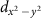and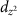components split into two A1 bands along Δ (or U). Finally, along Σ (or S), two A1 electron-like bands carryingandcomponents, respectively, display a linear dispersion and cross at a point denoted Q, located almost exactly at ɛF between the Γ and M points (or between Z and A).

The resulting Fermi surface consists of four sheets. The Eg band forms a hole pocket centred at Γ with a peculiar shape similar to that of a carambola fruit. As a result of the kz dispersion along Λ, this band forms an electron pocket centred at Z with square-shaped section. The Ag bands form a strongly oblated electron pocket centred at R. Finally, the two linearly dispersing A1 bands with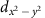andcomponent form a Dirac-like cone with elliptic-like section and hole character. The origin Q of the cone is located near kz=0. All pockets are small, which leads to semimetallic properties, as apparent from the pronounced dip of the DOS at ɛF, and to enhanced electronic correlations. Each band is at least twofold degenerate in the orbital sector since the glide reflection plane of the P4/nmm symmetry generates two Ni positions, r1=(1/4,1/4,z) and r2=(3/4,3/4,−z), in the unit cell.

### Angular resolved photoemission spectroscopy

To obtain high-quality spectra, we optimized the growth of BaNiS2 single crystals as described in the experimental methods section. We successfully measured ARPES spectra on several high-purity single crystals cleaved in situ at low temperature within the ab plane, which allowed us to measure the in-plane band dispersion, ɛk. As an indication of the improved purity of these crystals, resistivity measurements (see Methods section) yielded residual resistivity ratios RRR≈12–17 significantly larger than those 4 reported previously14,25. In Fig. 2, we show the 26 eV ARPES bands measured in both p- and s-polarizations along the Δ (or U) and Σ (or S) symmetry directions. Consistent with the orbital character of the bands calculated without SO coupling (Fig. 1c), due to the different parity of the d-orbitals with respect to the scattering plane, thestates are observed only in p-polarization along Σ, thestate in p-polarization along Δ and in s-polarization along Σ and the dyz and dxz states in both polarizations along Δ.

A quantitative comparison between experimental and calculated bands is shown in Fig. 3a, where the previous GGA+U bands are plotted together with the full ARPES band structure obtained by merging the p- and s-polarized spectra of Fig. 2. We noticed that the inclusion of the Hubbard repulsion U term in the GGA calculations improves significantly the agreement with the experiment, especially concerning the energy position of the B1g band at Γ (or Z) and the dispersion of the B1 band along Γ–X (or Z–R). To estimate the kz value, we calculated the band dispersion at different kz values, kz=0,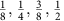(in crystal units of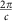), and best agreement with the experiment is obtained for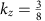. In Fig. 3a–c, the corresponding symmetry points and lines are labelled using primed letters.

As predicted by the calculations for, the experimental Fermi surface of Fig. 3b consists of the four hole pockets of the Dirac-like bands along S' and of the square-shaped electron pocket centred around Z'. A slight discrepancy between experiment and calculations concerns the hole pocket centred at R', not seen experimentally. According to the calculations, this may suggest that the kz value probed by the 26 eV photons rather lies in the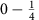kz range. In fact, in the calculated Fermi surface of Fig. 3d, both hole pockets at Z and at R shrink rapidly with decreasing kz, so an accurate determination of the relative kz position of the bottom of these pockets goes beyond the capabilities of the present DFT method. In summary, the experimental data are explained by a pocket at R located at a higher kz value than calculated. This explanation is supported by the fact that the 100 ev ARPES data do probe the bottom of the pocket, as seen in Fig. 4b. We conclude that the 26 eV data of Figs 2, 3 and the 100 eV data of Fig. 4b probe the bands in the vicinity ofand of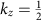, respectively.

## Discussion

We are now in the position of analysing one of the main features of the band structure, that is, the large ≈50 meV splitting of the Eg bands at Z, which suggests the importance of SO coupling effects not included in the calculations. A straightforward symmetry analysis24,26 substantiates this hypothesis. As far as the SO band splittings is concerned, we consider the k-points with, such as Z, on the same footing as the k′ points with, such as Z'. The reason is that the predictions of splitting for the little groups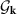of the former points are valid for the little groups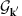of the latter points as well, asare subgroups of. Thus, the symmetry analysis of the SO band splitting is the same for both 26 eV and 100 eV ARPES bands shown in Figs 3a and 4b, respectively. This analysis shows that, while the Eg bands are degenerate in the non relativistic calculations, the product of their IR Z5 of the D4h little group for the Z symmetry point with the D1/2 spin IR yields the following sum of IR's:We have then recalculated the band structure including the SO coupling. Remarkably, the result shown in Figs 3a and 4a is fully consistent with the above band splitting. The SO coupling also accounts for the experimental observation of a mixing of the B1g band below the Fermi level with the lower split Eg band, clearly visible in the data at Z (Fig. 3a). Indeed, by denoting Z3 the IR of the B1g band at Z, one obtains, so the two bands are described by the same Z12 symmetry in the presence of SO coupling.

The exciting feature of BaNiS2 revealed by the present work is that, in addition to the ordinary SO splittings discussed above, both the experimental bands and those calculated with SO coupling display Rashba splittings, as shown in Fig. 4a. We focus on the splitting of the electron-like band with A1 character withandcomponents located just above ɛF near R along T (or near X along Y) and of the hole-like band located in the same k region ≈0.4 eV below ɛF. As seen in Fig. 1c, near R the latter band displays B1 and B2 character with dxz and dyz components, respectively, that progressively evolves into A1 character withcomponent moving to A. A close-up of the ARPES bands in Fig. 4b shows that only the bottom of the electron-like band is observed, as mentioned above; so the prediction of Rashba effect cannot be verified experimentally for this band but a slight electron doping may be sufficient to probe it. On the other hand, the predicted Rashba splitting is clear in the hole-like band. The splittings in wave vector and in energy are as large as Δk=0.2 Å−1 and Δɛ≈150 meV, respectively. The latter value is even larger than in BiTeI 9 or SrTiO3 (ref. 10), in spite of the absence of heavy elements or surfaces.

Both electron-like and hole-like bands are k split along R–A by the same Rashba interaction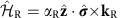, where kR=kk0 and k0 is the wave vector of the R point. While the electron-like upper band would be centred at R in the absence of SO correction, the hole-like band would have a maximum located between R and A. As shown by the present calculations, this difference leads to two different shapes of the spinor band structure, illustrated in Figs 4b and 5a, respectively. In Fig. 5a, the splitting of the electron-like band is decribed by the standard Mexican-hat shape. In Fig. 4b, the calculations predict a displaced split maximum for the hole-like band along the same direction, in agreement with the ARPES data. We fit the latter band using the band dispersion obtained by diagonalising the above Rashba Hamiltonian and find αR=0.26 eV Å from ARPES, in excellent agreement with the calculated value 0.28 eV Å. The difference between experimental and calculated splitting is due to the limited accuracy of the GGA+U approximation in calculating the unperturbed (that is, without SO) band dispersion and its mass renormalization. We computed the Rashba parameters also for the Mexican-hat splitting of the electron-like band, barely visible experimentally at ɛF. For this band, we obtained αR=0.19 eV Å, kR=0.04 Å−1 for the position of the minimum of the band with respect to R, and ɛR=11 meV for the depth of the Mexican hat (Fig. 5a).

Contrary to the usual Rashba case, here the spin degeneracy is not lifted, even for the electron-like Mexican-hat band, because every energy level is at least twofold degenerate due to the two-fold multiplicity of the Ni site. It follows that the Rashba Hamiltonian involves two—instead of one—spinors. Our calculations show (Fig. 5c) that the corresponding Bloch states,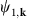and, are strongly localized either at r1 or at r2, where the dipolar crystal field (and the NiS5 pyramid) has opposite orientation, ±Ez, along the z direction. Owing to this localization, the Hamiltonian splits into two independent Rashba Hamiltonians, one for each position, with a staggered distribution of Rashba coupling αR forming a Rashba crystal in a square lattice. This situation corresponds to a LIA, as discussed by Zhang et al.11 who called hidden the resulting compensated spin polarization to distinguish it from that of the usual bulk inversion asymmetry and surface inversion asymmetry cases.

In the present case, the spin chirality at the two Ni positions, r1 and r2, is opposite, so the net spin polarization of the unit cell is zero. For the Bloch state at r1, where Ez>0, the solution for the Rashba eigenspinor at a given wave vector k readswhere the superscript ± denotes the upper and lower energy branch, respectively, and. Since Ez has opposite sign at r2, it follows that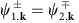; hence, each Rashba branch is made of two opposite spinors whose spatial part is assigned to one of the two Ni positions. This is illustrated in Fig. 5c, where the spatial and spin parts of both Bloch states are plotted in real space at k=(crystal units), where the splitting is largest. At this k point, φ=0, so, according to equation (2), the Rashba spin moment is oriented along the y direction with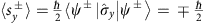. This picture is nicely confirmed by our ab initio calculations. The Bloch states projected on a given Ni position yield a local spin moment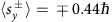for the two branches, while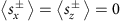. The slight reduction of sy with respect to the expected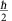value for the electron is due to the almost complete localization of each Bloch state around one Ni position (Fig. 5c). Finally, one notes that spin-chiral polarized states can be formed by applying a magnetic or electric field.

Using a point-like model for the S anion and for the Ba and Ni cations, the dipolar crystal field at the Ni site is estimated to be Ez≈1.4 V Å−1. This huge value accounts for the large Rashba effect observed and for the stability of the structure. Indeed, the staggered orientation of the NiS5 pyramids minimizes the Coulomb repulsion between apical sulphur atoms. The resulting staggered field configuration turns out to be a very effective LIA mechanism. Thus, the magnitude of the Rashba effect observed reflects the magnitude of the cohesive energy of the crystal. To justify quantitatively this picture, using the GGA–DFT method, we calculated the dependence of the Rashba coupling αR at R and the SO splitting ΔɛZ at Z as a function of the Ni position, z, along the c axis within the NiS5 pyramid. The result reported in Fig. 5b shows that the maximum values for both αR and Δɛz are obtained close to the equilibrium position of Ni, which means that the most stable structure corresponds to the largest Rashba splitting. This observation suggests an analogy with the Jahn-Teller systems where a gain of electronic energy drives a strain field that splits degenerate electronic states; here, the crystal field is the cause of the splitting.

Following Zhang et al.11, the present observation of Rashba effect in a centrosymmetric crystal arises from the LIA C4v at the Ni site caused by the above staggered field, which contrasts the usual case of Rashba band splitting at Γ in noncentrosymmetric crystals. The availability of a huge crystal field at the electronically active Ni site extends the possibilities of Rashba band engineering to light elements. The Rashba couplings obtained here could be further enhanced by substituting Ni for heavier 4d or 5d ions. To verify this expectation, we carried out preliminary GGA–DFT ab initio calculations on the hypothetical compounds BaPdS2 and BaPtS2 assuming that the crystal structure would be similar to that of BaNiS2. Our expectation is confirmed by a significant enhancement of the Rashba coupling for the electron-like band; namely, we obtain αR=0.35 and 0.73 eV Å for the relaxed structure of the Pd and Pt compound, respectively. The corresponding band splittings are also dramatically enhanced up to Δɛ≈ 0.5 eV in both compounds, which would enable a band structure engineering of solids in view of spintronics applications.

## Methods

### Single-crystal growth

High-purity BaNiS2 single crystals were synthesized by a self-flux method, as described elsewhere27,28. In brief, we first prepared a slightly off-stoichiometry mixture of finely grinded high-purity BaS (99.9%), Ni (99.999%) and S (99.9995%) powders in a graphite crucible. The chosen Ba:Ni:S=0.10:0.425:0.475 composition ensures the crystal growth through the liquidus line of the pseudobinary phase diagram BaNiS2-2/3 Ni3S2+δ system as shown in ref. 27. The crucible was then sealed under high vacuum at typical pressures P10−5 mbar in a silica tube, heated to 1,000 °C during 2 days and then quenched into water. The product was then grinded and pressed into a pellet for a second time by adding a 10% molar excess of sulphur and the aforementioned heat treatment was repeated. The silica tube was then slowly cooled from 1,000 °C down to 800 °C at a rate of 0.5–1 °C h−1 and then quenched into water. The as-prepared melt contains platelet-like shiny black crystals of typical size 1 × 1 × 0.1 mm3 that can be mechanically removed from the melt. The crystalline quality, chemical composition and impurity concentration were checked by means of single-crystal X-ray diffraction, energy dispersive X-ray analysis and resistivity measurements in the van der Pauw configuration.

### Angular resolved photoemission spectroscopy

The ARPES experiment was carried out on crystals freshly cleaved in situ within the ab-plane at 100 K in ultra-high vacuum, at pressures of 10−11 mbar or better, at the BaDElPh beamline of the Elettra synchrotron in Trieste, Italy, and at the Cassiopée beamline of the SOLEIL synchrotron in Saint-Aubin, France. In the former experiment, we used an incident photon energy hv=26 eV with a Δɛ=5 meV photon energy resolution. To determine the parity of the bands, the experiment was performed in both p and s photon polarizations. In the former case, the incoming electric field, perpendicular to the photon momentum, lies within the scattering plane defined by the photon momentum and by the photoelectron momentum. In the latter case, the electric field is perpendicular to the scattering plane. In our geometry, the p polarization forms an angle of 45° with the ab-plane when at normal emission, while the s polarization is parallel to this plane. In the experiment conducted at SOLEIL, the photon energy was 100 eV and the photon energy resolution was Δɛ=10 meV.

### Ab initio calculations

The ab initio calculations have been carried out in the GGA–DFT framework with the Perdew–Burke–Ernzerhof functional, implemented in the Quantum ESPRESSO package29. In the GGA+U calculations, we used the rotationally invariant formalism30 with the Hubbard matrix correlating the Ni 3d orbitals and fulfilling the atomic spherical symmetries. The Hubbard parameters are U=3 eV, J=0.95 eV, and the F4/F2 Slater integral ratio is taken equal to the atomic value. Ni, Ba and S atoms are described by norm-conserving pseudopotentials. The Ni pseudopotential has 10 valence electrons (4s2 3d8) and non linear core corrections. For Ba, the semi-core states have been explicitely included in the calculations. The S pseudopotential is constructed with the 3s2 3p4 in-valence configuration. In the GGA+U calculations with SO coupling, the Ni pseudopotential is fully relativistic and a non-collinear spin (spinor) formalism is used in the DFT framework. The geometry of the cell and the internal coordinates are taken from the experimental values reported previously14. The plane-wave cutoff is 120 Ry for the wave function and 500 Ry for the charge. A 8 × 8 × 8 electron-momentum grid and a Methfessel–Paxton smearing of 0.01 Ry are used in the electronic integration during the self-consistent loop. Further calculations with a 16 × 16 × 16 electron-momentum grid and tetrahedra interpolation have been performed for a more accurate determination of the Fermi level starting from a previously converged 8 × 8 × 8 self-consistent electron density. For band structure and Fermi surface calculations, we carried out a Wannier interpolation of the ab initio band structure by means of the Wannier90 (ref. 31) program on a Nw=4 × 4 × 4 electron-momentum mesh by including all Ni d and S p states in a 11 eV energy window, which allows to obtain highly accurate interpolated bands.